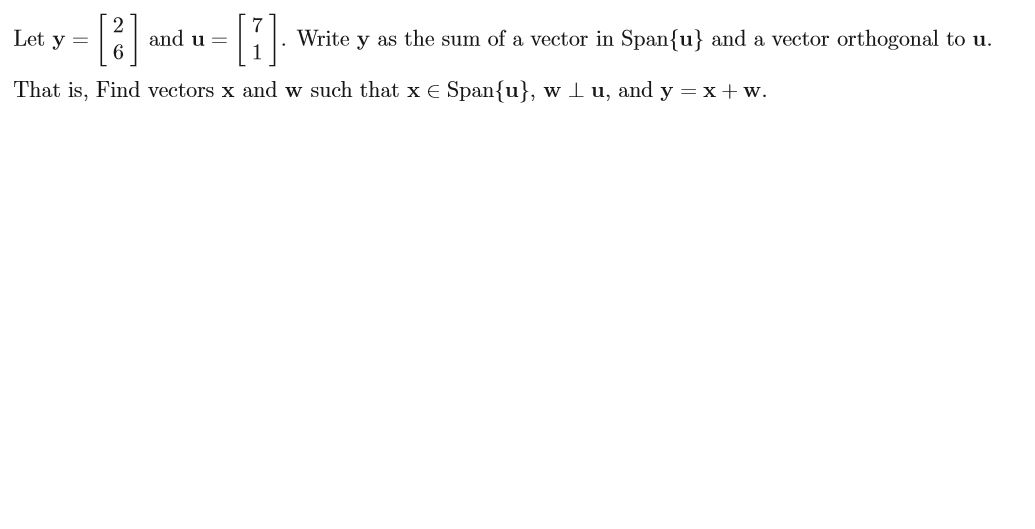# Write y as the sum of a vector in Spanfu} and a vector orthogonal to uand uLet y =That is, Find vectors x and w such that x E Spanfu, w l u, and y xw

Question
23 views

Linear algebrahelp_outlineImage TranscriptioncloseWrite y as the sum of a vector in Spanfu} and a vector orthogonal to u and u Let y = That is, Find vectors x and w such that x E Spanfu, w l u, and y xw fullscreen
check_circle

Step 1

From the given information:

Let,

Step 2

It is required to calculate y as sum of two vectors that is y = x + w such that x belongs to        span {u} and w is orthogonal to u.

So, first calculate:

Step 3

Now, put the values in t...

### Want to see the full answer?

See Solution

#### Want to see this answer and more?

Solutions are written by subject experts who are available 24/7. Questions are typically answered within 1 hour.*

See Solution
*Response times may vary by subject and question.
Tagged in

### Math